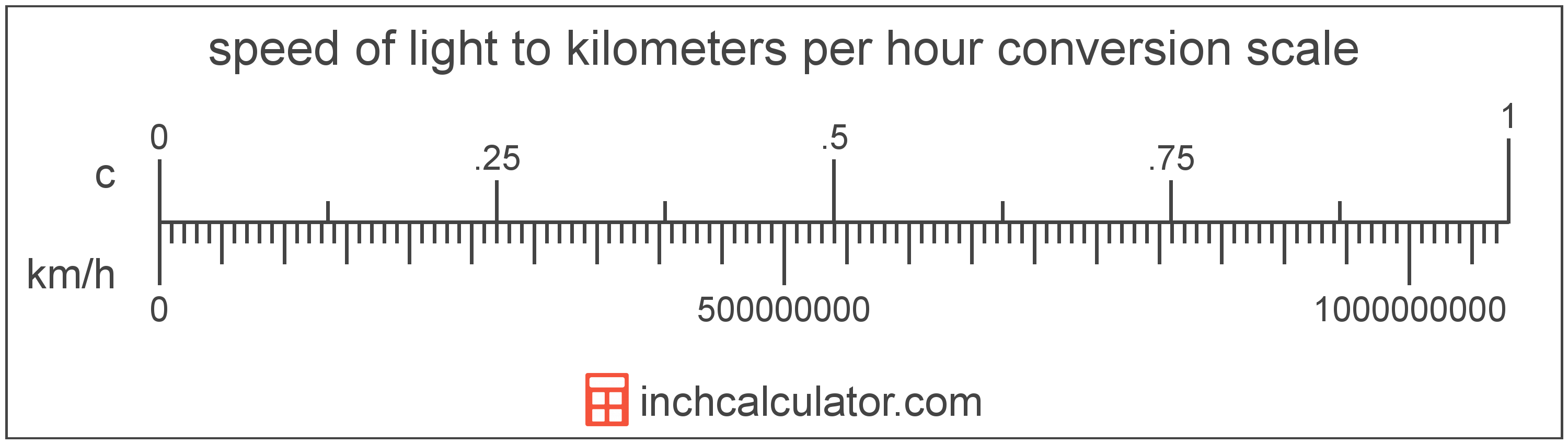# Speed Of Light to Kilometers Per Hour Conversion

Enter the speed in speed of light below to get the value converted to kilometers per hour.

Results in Kilometers Per Hour:1 c = 1,079,252,848.7999 km/h

## How to Convert Speed Of Light to Kilometers Per HourTo convert a speed of light measurement to a kilometer per hour measurement, multiply the speed by the conversion ratio.

Since one speed of light is equal to 1,079,252,848.7999 kilometers per hour, you can use this simple formula to convert:

kilometers per hour = speed of light × 1,079,252,848.7999

The speed in kilometers per hour is equal to the speed of light multiplied by 1,079,252,848.7999.

For example, here's how to convert 5 speed of light to kilometers per hour using the formula above.
5 c = (5 × 1,079,252,848.7999) = 5,396,264,243.9996 km/h

Speed of light and kilometers per hour are both units used to measure speed. Keep reading to learn more about each unit of measure.

## Speed Of Light

The speed of light is equal to exactly 299,792,458 meters per second, or 670,616,629 miles per hour. The definition of the speed of light is actually derived from the most recent 1983 international definition of the meter.

Speed of light can be abbreviated as c; for example, 1 speed of light can be written as 1 c.

## Kilometers Per Hour

Kilometers per hour are a measurement of speed expressing the distance traveled in kilometers in one hour.

The kilometer per hour, or kilometre per hour, is an SI unit of speed in the metric system. Kilometers per hour can be abbreviated as km/h, and are also sometimes abbreviated as kph. For example, 1 kilometer per hour can be written as 1 km/h or 1 kph.

In formal expressions, the slash, or solidus (/), is used to separate units used to indicate division in an expression.

Kilometers per hour can be expressed using the formula:
vkm/h = dkmthr

The velocity in kilometers per hour is equal to the distance in kilometers divided by time in hours.

## Speed Of Light to Kilometer Per Hour Conversion Table

Speed of light measurements converted to kilometers per hour
Speed Of Light Kilometers Per Hour
0.000000001 c 1.0793 km/h
0.000000002 c 2.1585 km/h
0.000000003 c 3.2378 km/h
0.000000004 c 4.317 km/h
0.000000005 c 5.3963 km/h
0.000000006 c 6.4755 km/h
0.000000007 c 7.5548 km/h
0.000000008 c 8.634 km/h
0.000000009 c 9.7133 km/h
0.0000000001 c 0.107925 km/h
0.000000001 c 1.0793 km/h
0.00000001 c 10.79 km/h
0.0000001 c 107.93 km/h
0.000001 c 1,079 km/h
0.00001 c 10,793 km/h
0.0001 c 107,925 km/h
0.001 c 1,079,253 km/h
0.01 c 10,792,528 km/h
0.1 c 107,925,285 km/h
1 c 1,079,252,849 km/h

## References

1. National Institute of Standards and Technology, SI Redefinition: Meter, https://www.nist.gov/si-redefinition/meter
2. National Institute of Standards and Technology, NIST Guide to the SI, Chapter 6: Rules and Style Conventions for Printing and Using Units, https://www.nist.gov/pml/special-publication-811/nist-guide-si-chapter-6-rules-and-style-conventions-printing-and-using